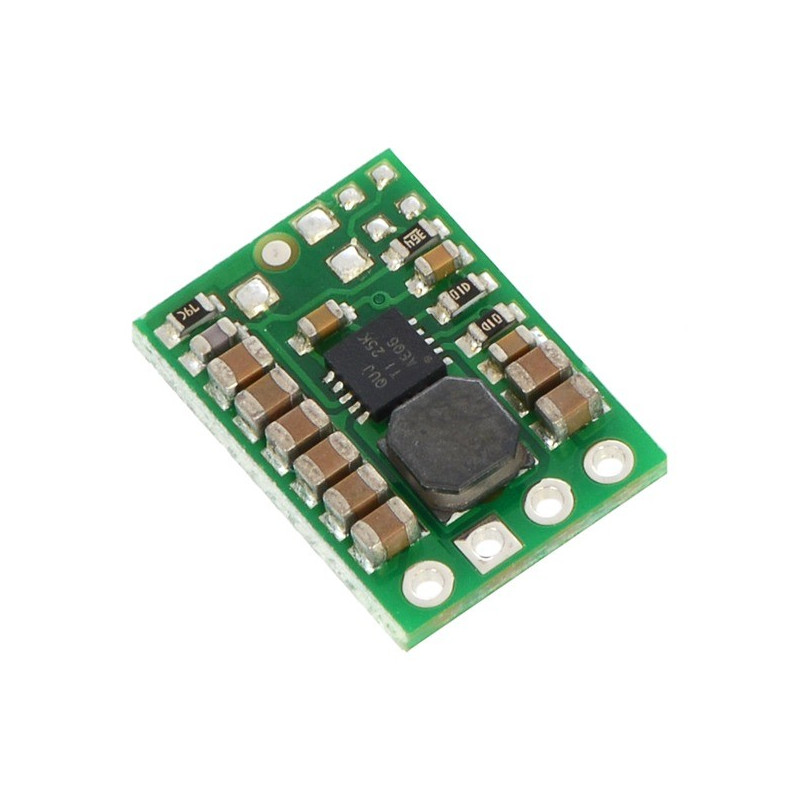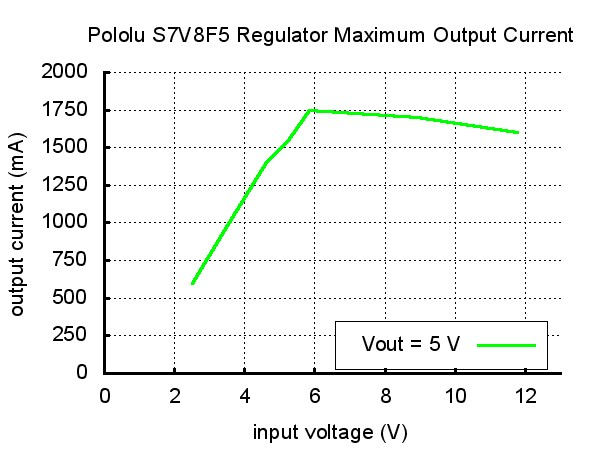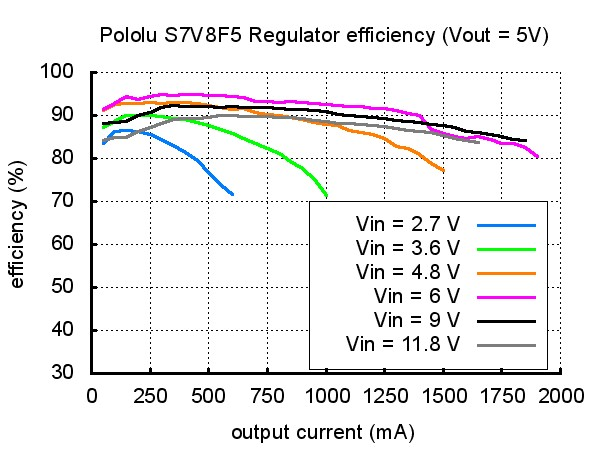Maximize

# Step-Up/Step-Down Voltage Regulator S7V8F5 - 5V 1A - Pololu 2123

Converter module pulse power. Input voltage 2.7 V to 11.8 V. Output voltage: 5 V Maximum output current: 1 A. Dimensions: 11.5 x 16.5 x 2.54 mm

PLL-01428

Pololu

Dodano produkt do koszyka

Price 23,90 zł

## Description of product: S7V8F5 Converter, step-up/step-down 5V 1A

S7V8F3 Converter is a switching regulator type voltage step-up/step-down (buck-boost). Converts an input voltage of 2.7 V and 11.8 V output 5V with an average efficiency of 80%. The input voltage can be below, above or equal to the input values, which makes the layout functional and versatile. Flexibility for input voltages, making the system works perfectly in systems powered by batteries, which require a stable voltage supply and its independence from the battery charge level.

## Examples of application

• Maintaining a stable voltage of 5V in a system operating with a basket with four NiMh batteries (fingers) that give a fully charged voltage of about. 6, a partially discharged below 5V.
• Power supply voltage 5V IC single cell Li-Po during the entire discharge cycle of the battery.
• Power chip 5V from 9V batteries that are partially discharged can give a voltage below what is needed.In the standard application system allows current up to 1A in step-down, i.e. when the supply voltage is higher than porządane output voltage. When the input voltage is below the output mode (step-up), the maximum output current of the Converter decreases in accordance with the schedule posted in the picture below.The graph shows the dependence of the maximum power load from the input voltage.

## Method of application

The module has four pins: input voltage VIN, output voltage VOUT, ground to GND and pin SHDN of switching the system to standby state.

• VIN - input voltage from 2.7 V to 11.8 V. When the lower voltage of the inverter does not start. Above, can eventually damage the chip.
• GND - the ground.
• VOUT - output voltage 5 V
• SHDN - input low (<0.4 v="" puts="" the="" system="" into="" low="" power="" mode="" current="" consumption="" in="" sleep="" depends="" on="" resistor="" 100="" ohms="" podci="" gaj="" cego="" shdn="" to="" vin="" and="" is="" 10="" ua="" per="" volt="" for="" example="" if="" voltage="" 5="" circuit="" will="" consume="" value="" of="" 50="" li="">

Connectors respectively signed on the Board. Terminal pitch is 2.54 mm (popular goldpin connectors). The module can be mounted on the contact plate, to connect with wires or solder in a special printed circuit Board.

## The efficiency of the Converter

Efficiency is defined as the ratio of input power to output power (Pout/Pin). This parameter is of particular importance when running on battery power when it is important that the system worked as long as possible on a single charge. The efficiency of the Converter depends on the strength of the current flow and the input voltage (chart below). On average 80-95%, allowing you to use almost the maximum energy from the battery.The fitness of a controller for the output voltage to 5V.

## Jumping voltages (pins)

In electronic circuits, the current run can lead to the emergence of so-called pins, that is, sharp voltage spike to a value above a certain level. If the amplitude of the pin exceeds the permissible value of the controller, it can fail. Therefore, if the system will be powered with voltage not higher than 9 V or the load will have high inductance, we highly recommend you wlutowanie capacitor value of 33uF or higher, as close as possible to the system between VIN and GND.

## Specification of the Converter Pololu S7V8F5

• Input voltage: from 2.7 V to 11.8 V
• Maximum output current:
• 1 A (step-down)
• 0.5 A (step-up)
• Output voltage: 5 V
• Temperature protection
• The short-circuit protection
• Dimension: 11.5 x 16.5 x 2.54 mm
• Weight: 0.6 g (without connectors)

• Length 17 mm
• Width 12 mm
• Height 3 mm
• Voltage to 2.7 V
• Voltage from 11.8 V
• Voltage output from 5.0 V
• Output voltage to 5.0 V
• Nominal output voltage 5.0 V
• Current 1 A
• Stabilizer - Type2 switching
• Stabilizer - Type step-up/down

SAME DAY SHIPPING

Posting the payment for the order on our account on a business day up to 13:00 means sending the goods on the same day!

The table presents available forms of delivery in Poland

Carrier and
regulations

Bank wire
transfer

Payment
by cash

Delivery
time

Poczta Polska

9,90 PLN17,90 PLN1-3 days

Kurier GLS

14,90 PLN22,90 PLN

1-2 days